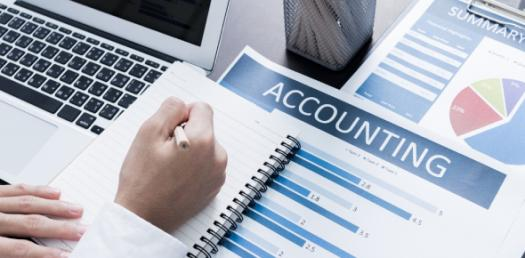# Quiz: Accounting Principle Trivia Questions

20 QuestionsSettingsWelcome to the Accounting principle trivia questions. Every person who is studying and want to become a practicing accountant. Then it is expected to know the basic accounting principles by heart as they serve as a guideline on how they should perform their job. Do give it a try and see just how well you understand them in practice. All the best!   ; &nbs p; &nb sp; &n bsp;

Related Topics
• 1.
The main purpose of cost accounting is to
• A.

A. maximise profits

• B.

B. help in inventory valuation

• C.

C. provide information to management for decision-making

• D.

D. aid in the fixation of selling prices.

• 2.
One of the most important tools in cost planning is
• A.

A. direct cost

• B.

B. budget

• C.

C. cost sheet

• D.

D. marginal costing.

• 3.
The purpose of financial accounting is to provide information for —
• A.

A. fixing prices

• B.

B. cost control

• C.

C. locating factory leading to wastages and losses

• D.

D. assessing the profitability and financial position of the firm.

• 4.
Fixed cost per unit increases when —
• A.

A. variable cost per unit increases

• B.

B. variable cost per unit decreases

• C.

C. production volume increases

• D.

D. production volume decreases.

• 5.
Variable cost per unit
• A.

A. varies when output varies

• B.

B. remains constant

• C.

C. increases when output increases

• D.

D. decreases when output decreases.

• 6.
Increase in total variable cost is due to
• A.

A. increase in fixed cost

• B.

B. increase in sales

• C.

C. increase in production.

• 7.
An example of variable cost is
• A.

A. property taxes

• B.

B. interest on capital

• C.

C. direct material cost

• D.

D. depreciation of machinery.

• 8.
An example of fixed cost is
• A.

A. direct material cost

• B.

B. works manager’s salary

• C.

C. depreciation of machinery

• D.

D. chargeable expenses.

• 9.
Cost accounting concepts include all of the following except.
• A.

A. planning

• B.

B. controlling

• C.

C. profit sharing

• D.

D. product costing.

• 10.
The three major elements of product costs are all but
• A.

A. direct materials

• B.

• C.

C. direct labour

• D.

D. indirect labour.

• 11.
Cost of goods produced includes
• A.

A. production cost and finished goods inventory

• B.

B. production cost and work-in-progress

• C.

C. production cost, work-in-progress and finished goods inventory.

• 12.
Cost of goods sold includes
• A.

A. cost of production and work-in-progress

• B.

B. cost of production and finished goods inventory

• C.

C. cost of production, work-in-progress and finished goods inventory.

• 13.
An overstatement of work-in-progress at the end of a period will
• A.

A. overstate cost of goods produced

• B.

B. understate current assets

• C.

C. understate gross profit

• D.

D. overstate net profit

• 14.
Conversion cost is equal to the total of
• A.

A. material cost and direct wages

• B.

B. material cost and indirect wagesc.

• C.

C. direct wages and factory overhead

• D.

D. material cost and factory overhead

• 15.
Multiple costing method is used in
• A.

A. oil refinery

• B.

B. car manufacturing company

• C.

C. sugar mill

• D.

D. multi-product company.

• 16.
Toy manufacturing company should use
• A.

A. unit costing

• B.

B. job costing

• C.

C. batch costing

• D.

D. multiple costing

• 17.
Electricity generating company should employ
• A.

A. unit costing

• B.

B. process costing

• C.

C. operation costing

• D.

D. operating costing.

• 18.
Job costing is used in
• A.

A. paper mills

• B.

B. chemical worksc.

• C.

C. printing press

• D.

D. textile mill.

• 19.
Output costing is employed in
• A.

A. mining quarries

• B.

B. steel production

• C.

C. brick kilns

• D.

D. all of these

• 20.
Contract costing is used in
• A.

A. ship-building

• B.

B. aeroplane industries

• C.

C. automobile industries.

• D.

D. None of these.